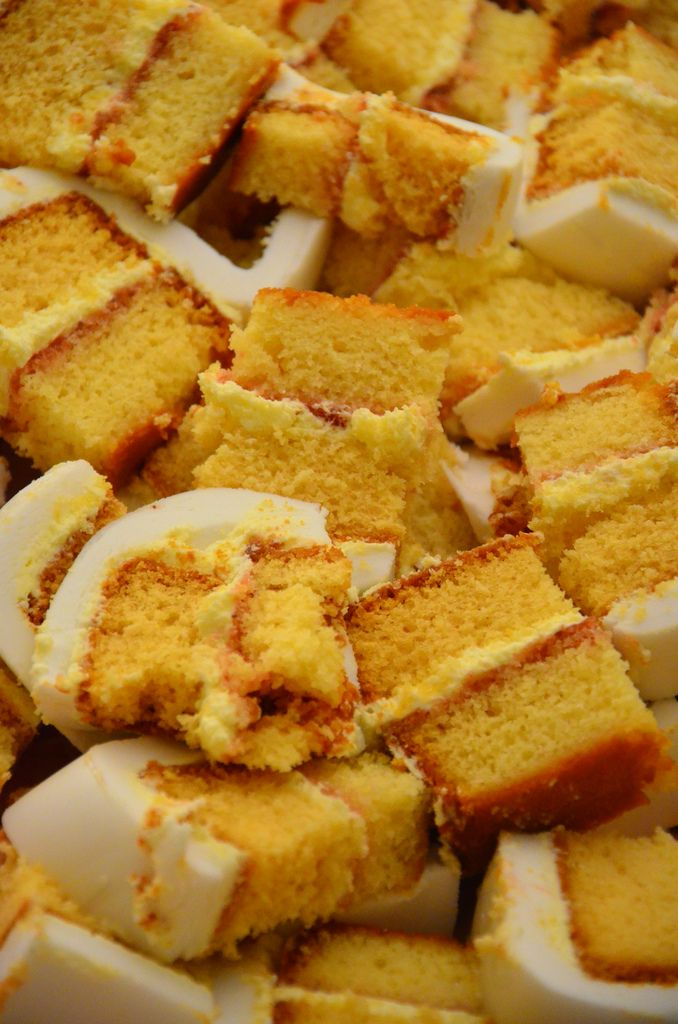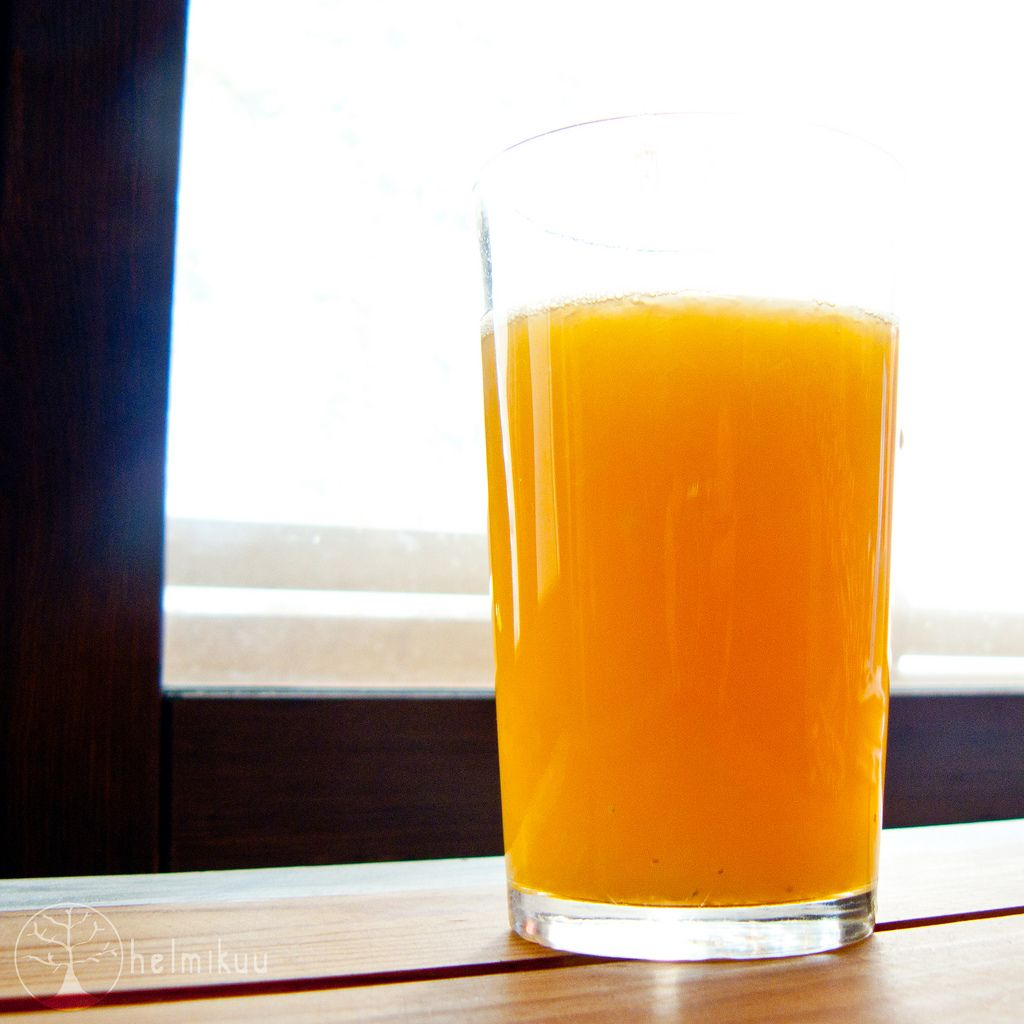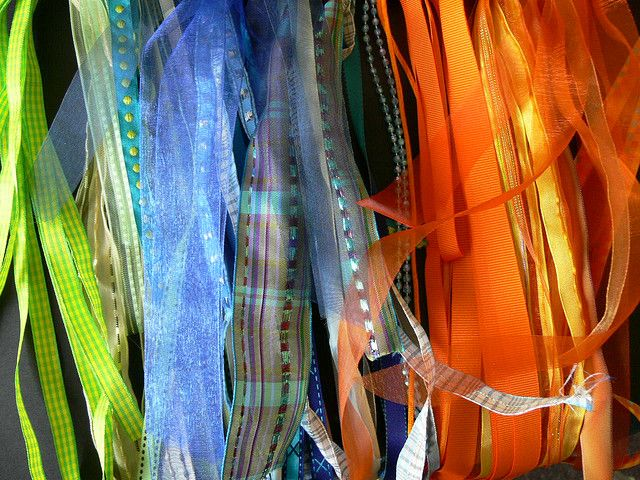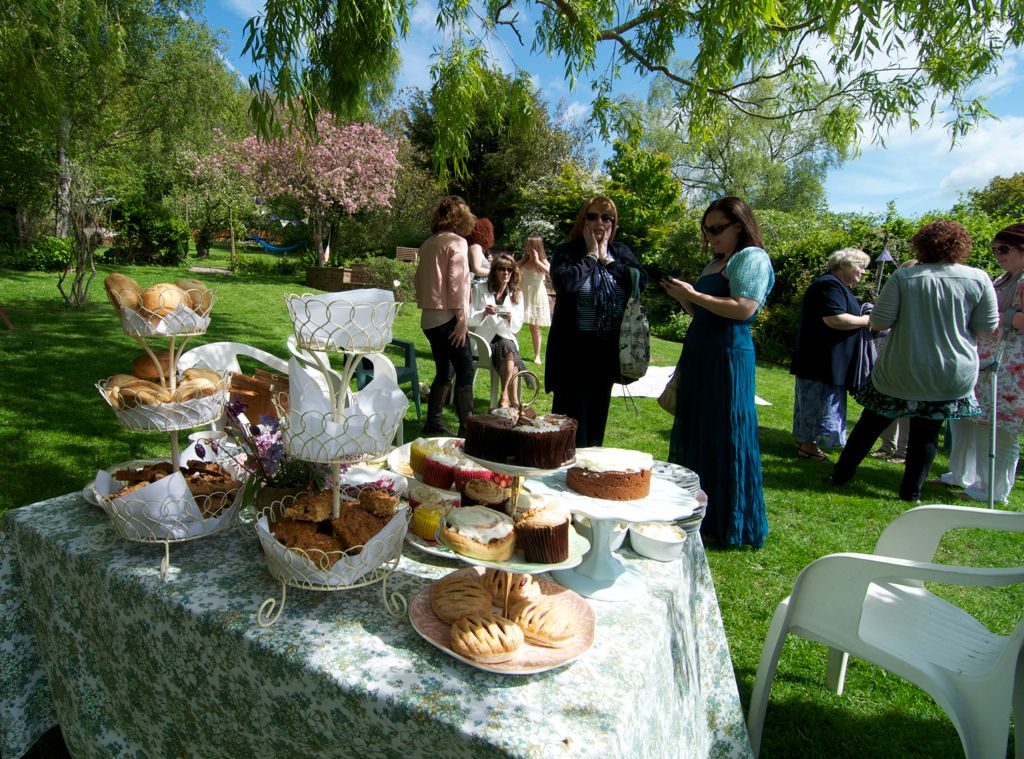Home Practice
For learners and parents For teachers and schools
Textbooks
Full catalogue
Pricing SupportLog in

We think you are located in United States. Is this correct?

# End of chapter activity

Exercise 5.9

The Grade 10 class at Masibambane High School are organising a tea party for the residents of a nearby old age home.

Some of the class members are going to bake cake, using the following recipe: $$\text{1}$$ cup butter $$\text{1}$$ cup sugar $$\text{1}$$ teaspoon vanilla essence $$\text{1}$$ teaspoon lemon extract $$\text{5}$$ eggs $$\text{2}$$ cups flour $$\text{1}$$ teaspoon baking powder $$\text{2}$$ egg whites for icingThe learners know that this recipe will make one cake, and that one cake yields $$\text{8}$$ slices. If there will be $$\text{60}$$ people at the tea party, and everyone has only one slice of cake, how many cakes do they need to make?

$$\text{60}$$ $$\div$$ $$\text{8}$$ = $$\text{7,5}$$ cakes. They can't bake half a cake so they will have to bake $$\text{8}$$.

The learners decide to bake $$\text{9}$$ cakes, so that there will be more than enough cake to go around.

How many cups of butter will they need?

$$\text{9}$$ cups.

How many litres of flour will they need? ($$\text{1}$$ cup = $$\text{250}$$ $$\text{ml}$$).

$$\text{1}$$ cake requires $$\text{2}$$ cups = $$\text{250}$$ $$\text{ml}$$ of flour. $$\text{9}$$ $$\times$$ $$\text{250}$$ $$\text{ml}$$ = $$\text{2 250}$$ $$\text{ml}$$ = $$\text{2,25}$$ litres

How many ml of vanilla essence will the learners need for $$\text{9}$$ cakes? ($$\text{1}$$ tsp = $$\text{5}$$ $$\text{ml}$$).

$$\text{1}$$ cake requires $$\text{1}$$ tsp = $$\text{5}$$ $$\text{ml}$$. $$\text{9}$$ $$\times$$ $$\text{5}$$ $$\text{ml}$$ = $$\text{45}$$ $$\text{ml}$$ of vanilla essence.

If vanilla essence is sold in $$\text{25}$$ $$\text{ml}$$ bottles, how many bottles will the learners need to buy?

$$\text{45}$$ $$\text{ml}$$ $$\div$$ $$\text{25}$$ = $$\text{1,8}$$ bottles. They can't buy $$\text{0,8}$$ of a bottle so they will have to buy $$\text{2}$$ bottles.

If vanilla essence costs $$\text{R}\,\text{7,85}$$ a bottle, how much will the required vanilla essence for $$\text{9}$$ cakes cost?

$$\text{2}$$ $$\times$$ $$\text{R}\,\text{7,85}$$ = $$\text{R}\,\text{15,70}$$

How many eggs will the learners need for $$\text{9}$$ cakes?

$$\text{5}$$ eggs $$\times$$ $$\text{9}$$ cakes = $$\text{45}$$ eggs.

If eggs are sold in boxes of $$\text{6}$$, how many boxes will they need to buy and how many eggs will be left over?

$$\text{45}$$ $$\div$$ $$\text{6}$$ = $$\text{7,5}$$ boxes. They can't buy $$\text{0,5}$$ of a box so they will have to buy $$\text{8}$$ boxes. $$\text{6}$$ $$\times$$ $$\text{8}$$ boxes = $$\text{48}$$ eggs. $$\text{48}$$ - $$\text{45}$$ = $$\text{3}$$ eggs left over.

If one box of eggs costs $$\text{R}\,\text{8,40}$$, how much will enough eggs for $$\text{9}$$ cakes cost?

$$\text{R}\,\text{8,40}$$ $$\times$$ $$\text{8}$$ boxes = $$\text{R}\,\text{67,20}$$

The cakes are baked in square cake tins. If the width of one cake is $$\text{200}$$ $$\text{mm}$$, how wide will $$\text{6}$$ cakes packed side by side be? (Give your answer in cm).

$$\text{200}$$ $$\text{mm}$$ $$\times$$ $$\text{6}$$ = $$\text{1 200}$$ $$\text{mm}$$ = $$\text{120}$$ $$\text{cm}$$

If one cake weighs $$\text{700}$$ $$\text{g}$$, and there are $$\text{8}$$ slices per cake, calculate how much one slice will weigh.

$$\text{700}$$ $$\text{g}$$ $$\div$$ $$\text{8}$$ = $$\text{87,5}$$ $$\text{g}$$

The cake needs to be baked in the oven for $$\text{40}$$ minutes at $$\text{180}$$$$\text{°C}$$. According to the oven's temperature dial, the temperature in the oven is $$\text{200}$$$$\text{°C}$$.

By how much must the oven cool before the cake can be baked?

$$\text{200}$$$$\text{°C}$$ - $$\text{180}$$$$\text{°C}$$ = $$\text{20}$$$$\text{°C}$$

What do you think will happen if they leave the oven temperature at $$\text{200}$$$$\text{°C}$$ and bake the cake at this higher temperature?

The cake will flop, or it may burn.

Other learners in the class are going to make fruit juice for the tea party.

They estimate that each person will have $$\text{600}$$ $$\text{ml}$$ of juice. How many litres of juice will they need to make in total (for $$\text{60}$$ people)?

$$\text{600}$$ $$\text{ml}$$ $$\times$$ $$\text{60}$$ = $$\text{36 000}$$ $$\text{ml}$$ = $$\text{36}$$ litres.

The class makes $$\text{40}$$ litres of juice. They wish to transport it in $$\text{1,5}$$ $$\ell$$ flasks.

How many flasks will they need?

$$\text{40}$$ litres $$\div$$ $$\text{1,5}$$ litres = $$\text{26,67}$$ flasks. They can't have $$\text{0,67}$$ of a flask so they will need $$\text{27}$$ flasks.

If they only have $$\text{15}$$ flasks, (and can transport $$\text{15}$$ flasks at once from the school to the home) how many trips will they have to make to get all the fruit juice to the tea party?$$\text{27}$$ flasks $$\div$$ $$\text{15}$$ flasks = $$\text{1,78}$$ trips. They can't make $$\text{0,78}$$ of a trip so they iwll have to make $$\text{2}$$ trips.

The old age home has a kettle that can hold $$\text{1,7}$$ litres of water.

How many $$\text{200}$$ $$\text{ml}$$ cups of water can be poured from the kettle when it is full?

$$\text{1,7}$$ litres = $$\text{1 700}$$ $$\text{ml}$$. $$\text{1 700}$$ $$\text{ml}$$ $$\div$$ $$\text{200}$$ $$\text{ml}$$ = $$\text{8,5}$$. So they can fill $$\text{8}$$ full cups.

What percentage of $$\text{1,7}$$ litres is one $$\text{200}$$ $$\text{ml}$$ cup?

$$\frac{\text{200}\text{ ml}}{\text{1 700}\text{ ml}} = \text{0,12}$$ = $$\text{12}$$ percent

Alison measures the temperature of the water in the kettle (with a thermometer) to be $$\text{65}$$$$\text{°C}$$. She knows that the water will boil at $$\text{100}$$$$\text{°C}$$. How much hotter (in °C) does the water need to be in order to boil?

$$\text{100}$$$$\text{°C}$$ - $$\text{65}$$$$\text{°C}$$ = $$\text{35}$$$$\text{°C}$$

The class decide they also want to buy bags of sweets for their tea party. If one $$\text{250}$$ $$\text{g}$$ bag of jelly beans costs $$\text{R}\,\text{5,49}$$, calculate how much $$\text{3}$$ $$\text{kg}$$ of jelly beans will cost.

There are $$\text{4 250}$$ $$\text{g}$$ bags in $$\text{1}$$ $$\text{kg}$$, so $$\text{3}$$ $$\text{kg}$$ = $$\text{3}$$ $$\times$$ $$\text{4}$$ = $$\text{12}$$ bags. $$\text{12}$$ $$\times$$ $$\text{R}\,\text{5,49}$$ = $$\text{R}\,\text{65,88}$$

The learners estimate that each person will eat $$\text{300}$$ $$\text{g}$$ of biscuits.

If there are $$\text{60}$$ people attending the party, how many kg of biscuits should they buy?

$$\text{60}$$ $$\times$$ $$\text{300}$$ $$\text{g}$$ = $$\text{18 000}$$ $$\text{g}$$ = $$\text{18}$$ $$\text{kg}$$

If biscuits are only sold in $$\text{500}$$ $$\text{g}$$ boxes, how many boxes will they need to buy?

$$\text{18 000}$$ $$\text{g}$$ $$\div$$ $$\text{500}$$ $$\text{g}$$ = $$\text{36}$$ boxes.

If one box of biscuits costs $$\text{R}\,\text{3,99}$$, calculate how much the learners will have to spend to buy enough biscuits for everyone.

$$\text{36}$$ boxes $$\times$$ $$\text{R}\,\text{3,99}$$ = $$\text{R}\,\text{143,64}$$

The Grade 10 class want to hang ribbons in the room where they are going to host their tea party. They want to cut the ribbons into $$\text{600}$$ $$\text{mm}$$ lengths.

If they buy $$\text{5}$$ $$\text{m}$$ of ribbon, how many whole pieces of $$\text{600}$$ $$\text{mm}$$ ribbon will they be able to cut?

$$\text{5}$$ $$\text{m}$$ = $$\text{5 000}$$ $$\text{mm}$$. $$\text{5 000}$$ $$\text{mm}$$ $$\div$$ $$\text{600}$$ $$\text{mm}$$ = $$\text{8,33}$$. So they can cut $$\text{8}$$ whole pieces of ribbon.

If the ribbon costs $$\text{R}\,\text{6,99}$$ per metre, how much will the ribbon cost in total?$$\text{R}\,\text{6,99}$$ $$\times$$ $$\text{5}$$ $$\text{m}$$ = $$\text{R}\,\text{34,95}$$

The class checks the weather report for the week in which the want to have the party:

 Day Mon Tues Wed Thurs Fri Temperatures $$\text{15}$$/$$\text{17}$$ $$\text{14}$$/$$\text{19}$$ $$\text{18}$$/$$\text{23}$$ $$\text{19}$$/$$\text{26}$$ $$\text{17}$$/$$\text{20}$$

If they want to have the tea party outside in the home's gardens, on which day should the plan to do it? Give reasons for your answer.Thursday - the weather will be warmest for an outdoor event.

What is the lowest temperature predicted for the week?

$$\text{14}$$$$\text{°C}$$.

What is the highest temperature predicted for the week?

$$\text{26}$$$$\text{°C}$$.

What is the difference between the minimum and maximum temperatures forecast for the Tuesday?

$$\text{19}\text{°C} - \text{14}\text{°C} = \text{5}\text{°C}$$.